# O G = {1,-1, :,-i} let e=' Find : 1) Order - of e Order Order...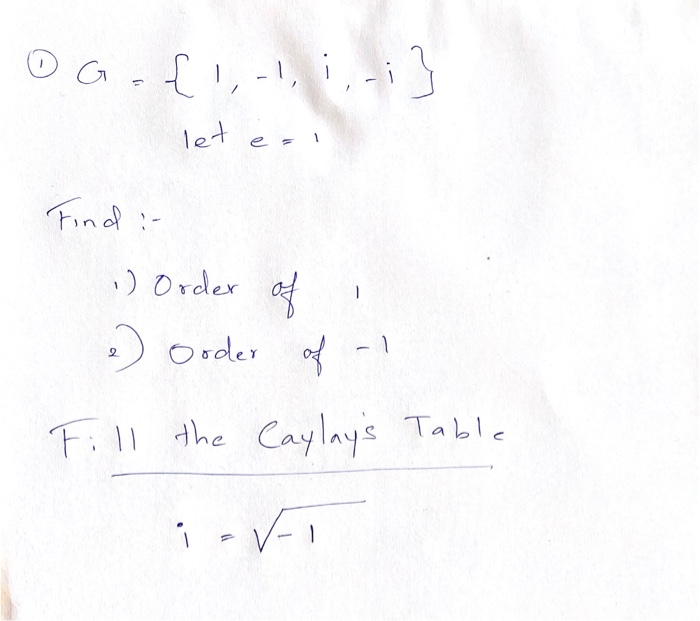O G = {1,-1, :,-i} let e=' Find : 1) Order - of e Order Order of Fill the Caylay's Table L' is .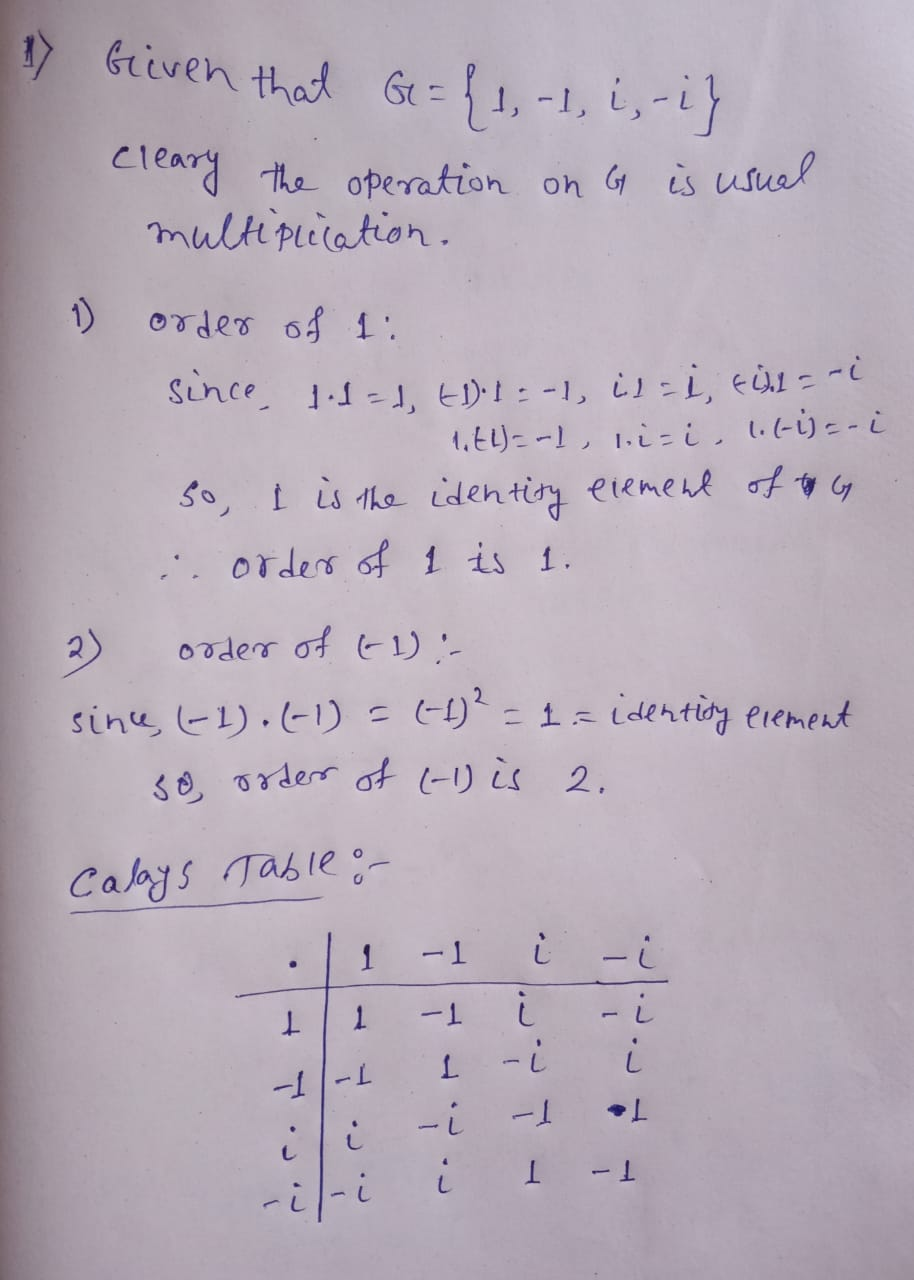##### Add Answer of: O G = {1,-1, :,-i} let e=' Find : 1) Order - of e Order Order...
Similar Homework Help Questions
• ### Let G be the following grammar: 1. S T 2. T O 3. T T 4. O V = E i [ E ] 5. V i 6. V i 7. E ( E) 8. E Construct the LR(0...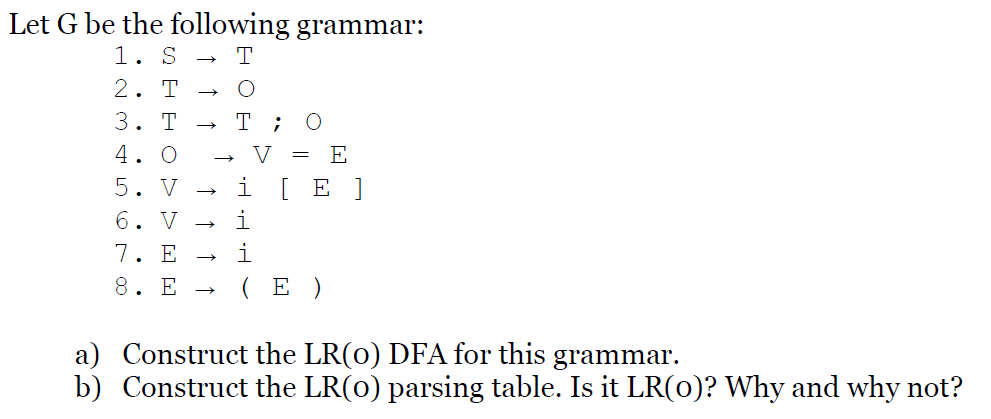Let G be the following grammar: 1. S T 2. T O 3. T T 4. O V = E i [ E ] 5. V i 6. V i 7. E ( E) 8. E Construct the LR(0) DFA for this grammar a) b) Construct the LR(0) parsing table. Is it LR(o)? Why and why not? Let G be the following grammar: 1. S T 2. T O 3. T T 4. O V = E i [ E...

• ### (9) Let G be a group, and let x E G have finite order n. Let...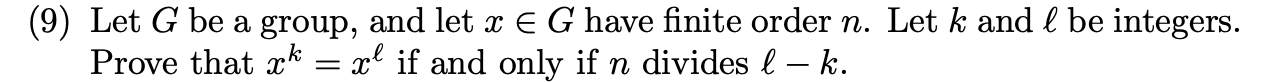(9) Let G be a group, and let x E G have finite order n. Let k and l be integers. Prove that xk = xl if and only if n divides l_ k.

• ### (1) Let (, A, /i) be a measure space = {AnE: A E A} is a o-algebra of E, contained in (a) Fix E E A. Prove that AE A. (...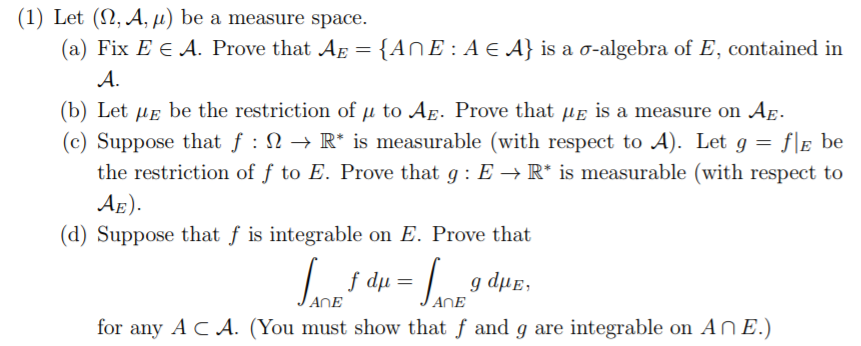(1) Let (, A, /i) be a measure space = {AnE: A E A} is a o-algebra of E, contained in (a) Fix E E A. Prove that AE A. (b) Let be the restriction of u to AE. Prove that uE is a measure on Ag (c) Suppose that f -> R* is measurable (with respect to A). Let g = f\e be the restriction of f to E. Prove that g E ->R* is measurable (with respect to...

• ### (1) Let (, A, i) be a measure space. {AnE: Ae A} is a o-algebra of E, contained in (a) Fix E E A. Prove that Ap = A. (b...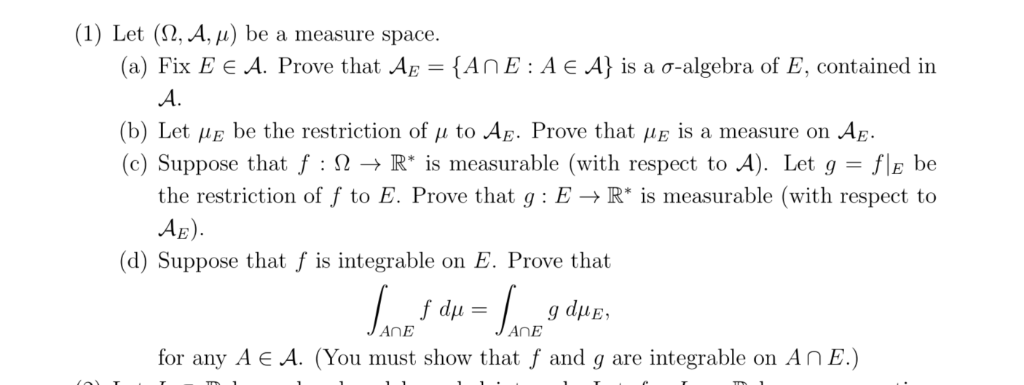(1) Let (, A, i) be a measure space. {AnE: Ae A} is a o-algebra of E, contained in (a) Fix E E A. Prove that Ap = A. (b) Let uE be the restriction of u to AĘ. Prove that iE is a measure on Ag. (c) Suppose that f : Q -» R* is measurable (with respect to A). Let g = the restriction of f to E. Prove that g : E ->R* is measurable (with respect...

• ### Let G be a finite group of order n. Let φ : G → G be the function given by φ(x) = z'n where rn E ...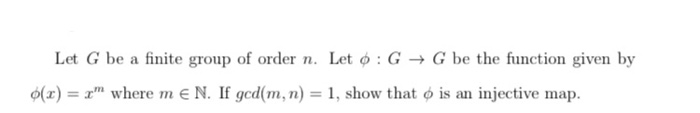Let G be a finite group of order n. Let φ : G → G be the function given by φ(x) = z'n where rn E N. If gcd(rn, n) = 1, show that φ s an injective map. Let G be a finite group of order n. Let φ : G → G be the function given by φ(x) = z'n where rn E N. If gcd(rn, n) = 1, show that φ s an injective map.

• ### Let , where and let . Find the order of the element in G/K.

Let , where and let . Find the order of the element in G/K.

• ### Let x be random variables with values a, b,c,d and e (in increasing order ) and...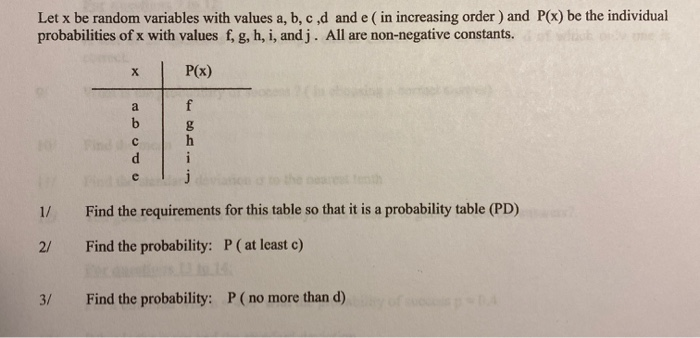Let x be random variables with values a, b,c,d and e (in increasing order ) and P(x) be the individual probabilities of x with values f, g, h, i, and j. All are non-negative constants. P(x) 1/ Find the requirements for this table so that it is a probability table (PD) 21 Find the probability: P (at least c) 3/ Find the probability: P ( no more than d)

• ### Problem 6 (20 points). Let G- (V,E) be a directed Let E' be another set of edges on V with edge l...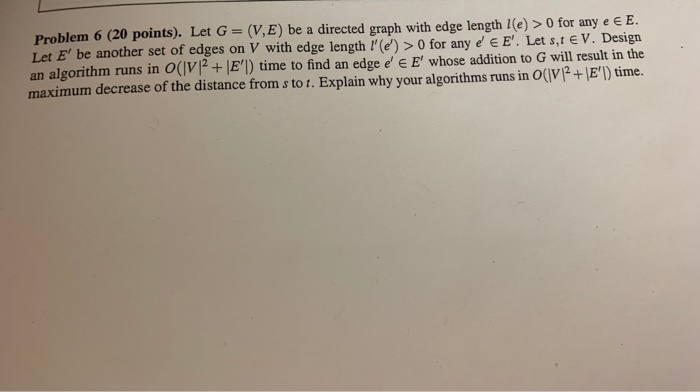Problem 6 (20 points). Let G- (V,E) be a directed Let E' be another set of edges on V with edge length '(e) >0 for any e EE. Let s,t EV. Design an algorithm runs in O(lV+ E) time to find an edge e'e E' whose addition to G will result in the maximum decrease of the distance from s to t. Explain why your algorithms runs in O(V2+E') time. graph with edge length l(e) >0 for any e E...

• ### Let G be a group of order 6 and let X be the set (a, b,c) E G3: abc That is, X is the set of trip...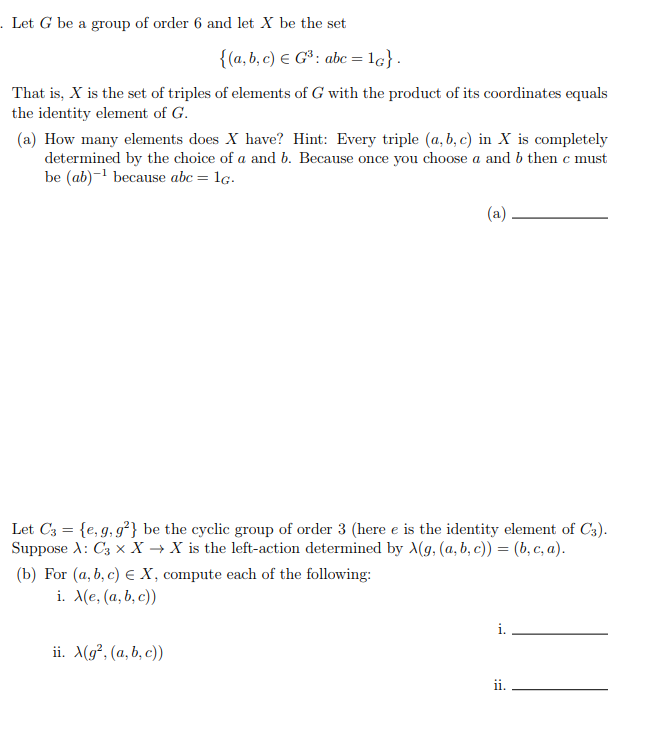Let G be a group of order 6 and let X be the set (a, b,c) E G3: abc That is, X is the set of triples of elements of G with the product of its coordinates equals the identity element of G (a) How many elements does X have? Hint: Every triple (a, b, c) in X is completely determined by the choice of a and b. Because once you choose a and b then c must be (ab)-1...

• ### 9S Let A I - O I - 0 1 -I Find A (a) Cb) Find...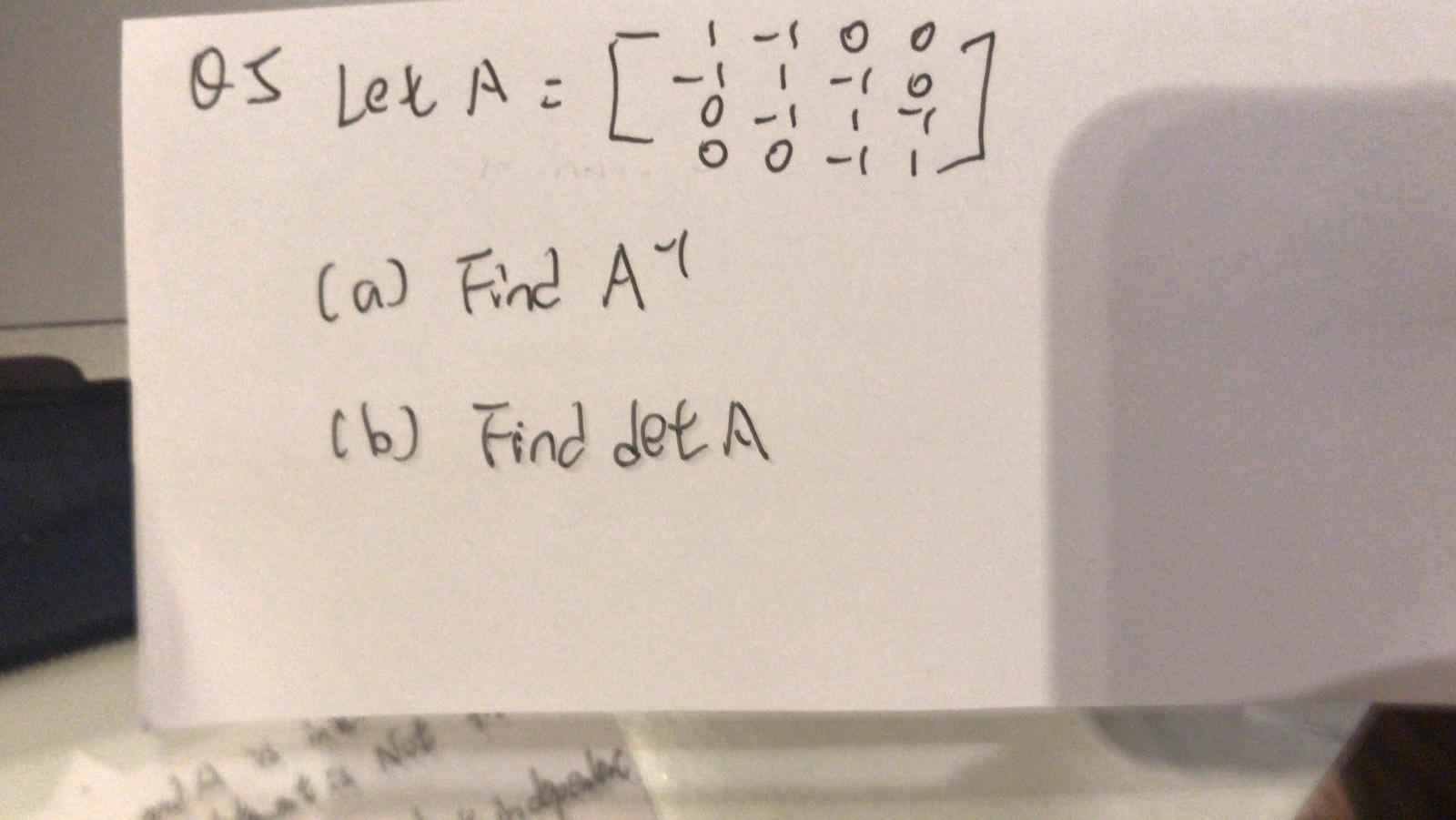9S Let A I - O I - 0 1 -I Find A (a) Cb) Find det A f aNot -700

Free Homework App